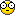SB Physics Help Room Sucks!
Would you like to react to this message? Create an account in a few clicks or log in to continue.

# Maple TA 23_2##Maple TA 23_2

1. A particle travels with 0.9357 c,where c is the speed of light, through the length of an experimenter's hall which is 41.14 m as measured by an observer who is stationary on the ground. What is the hall length for an observer "riding on the particle" (sheet 13,14') ? Indicate with a negative (positive) sign whether the "proper" length is (not) the one you measure when stationary in the hall.
=(41.14*(sqrt(1-(.9357)^2))) NEGATIVE

User X
Guest

##Maple TA 23_2

2. In "Relativity Country" the conductor in a train traveling with 8.145 x108 km/hr clocks the time it takes him to pass two markers on the ground to be 10.94 microseconds. What is the distance between the markers measured on the ground (sheet 13, calculate the distance measured by the conductor first)?
= Haven't solved this one completely yet, still working on solution

User X
Guest

##Maple TA 23_2

3. An electron moves with 88.76 % of the speed of light. By which factor is the moving mass larger than the restmass (sheet 17) ? Indicate with a negative (positive) sign whether the moving mass is infinite (finite) wenn you set the electron speed equal to the speed of light.
This one involves a little bit of work:
=(9.10938188E-28)/(sqrt(1-(.8876)^2))
=1.97765608E-27
NOT FINISHED YET
=(1.97765608E-27)/(9.10938188E-28)
=2.171010181 (NEGATIVE)
If you plug your numbers into the way I have mine set up as above, you will get the right answers, make sure your parenthesis are appropriate.

User X
Guest

##Maple TA 23_2

4. An electron (rest mass = 9.11x 10-31kg) has a speed of 0.9709 c, where c is the speed of light. What is its total energy (sheet 17,23) ? Indicate with a negative (positive) sign whether you use the rest mass (moving mass).
=Can't seem to get this one yet either....will update if I get them.

User X
Guest

##Maple TA 23_2

If anyone can provide any help with either #2 or 4, please help me out, I can't figure out where to go....I tried about 10 different things for both of them.....and if you can....please explain why, thanks.

User X
Guest

##quest 4

Okay for question 4 you first have to calculate the moving mass by using sheet 17. m=m_0/(sqrt((1-(speed given^2))
Where m_0 is the mass at rest which is given, and you are solving for m the moving mass.
The reason you use the speed given it beacause it is a number multiplied by c.

Now you must take the mving mass you just solved for and plug it tinto the total energy equation on sheet 23. E=mc^2
C is just 3E8 so c^2 is 9E16. To get this past just multiple the m you just solved for by 9E16. The answer is positive.

phyz
Guest

##question 2

Any ideas on number 2?

sck105
Guest

###2

First you have to find the distance measured by the conductor (I'm going to use the numbers from the posted question). We start by converting km/hr into m/s --> (8.145*10^11)/(3600) = 226250000 m/s ; Find d=v*t --> (226250000)*(10.94*10^-6) = 2475.175 m.
Now you use the formula "l_o=l/(√(1-(v^2/c^2)))" to solve for "proper" length --> 2475.175/(√(1-(226250000^2/((3*10^8 )^2))). Answer should be 3769.2? So you are really just using d=v*t and the "l" equation from sheet 13.

MAGGuest
Guest

##question 2

I've been trying question 2 so many times n I can't get it! can someone please helpp! thankss!

12345
Guest

##Re: Maple TA 23_2

look at the posted solution, what do you need help with? What are your numbers?

MAGGuest
Guest

12345
Guest

##question 2

i got it thanksss! but i don't understand how come the power is to the 11?? n not 8?

12345
Guest

##help i've been trying to do #2 since last week

In "Relativity Country" the conductor in a train traveling with 9.517 x108 km/hr clocks the time it takes him to pass two markers on the ground to be 11.51 microseconds. What is the distance between the markers measured on the ground (sheet 13, calculate the distance measured by the conductor first)?

k8
Guest

##4

can someone show work for #4
I don't get it at allplz help

studentx
Guest

##4

i got it nevermindstudentx
Guest

##ugh

finally i got a 100%. apparently you have to raise all you #s to 10^x not e^x. how do you know which one to use when?

k8
Guest

##Re: Maple TA 23_2

"e^x" is completely different from "E^x"... "E^x" is "10^x"

MAGGuest
Guest

##3

3. An electron moves with 88.76 % of the speed of light. By which factor is the moving mass larger than the restmass (sheet 17) ? Indicate with a negative (positive) sign whether the moving mass is infinite (finite) wenn you set the electron speed equal to the speed of light.
This one involves a little bit of work:
=(9.10938188E-2/(sqrt(1-(.8876)^2))
=1.97765608E-27
NOT FINISHED YET
=(1.97765608E-27)/(9.10938188E-2
=2.171010181 (NEGATIVE)
If you plug your numbers into the way I have mine set up as above, you will get the right answers, make sure your parenthesis are appropriate.

I'm not sure what number you're using for the mass of an electron. Isn't it 9.10938188E-31 kg? When I use that number in the equation I am required to multiply the final result by 10 in order to get the answer maple accepts, any idea what the deal with this is? Does the equation require non-SI units? (grams?)
Thanks

TI_83
Guest

##Question 3

To TI 83:

Doesn't matter if you use either 10^-28 or 10^-31, because you're finding a ratio. As long as you are consistent throughout the problem, you should get the same answer.

I used 9.11x10^-31 in my calculator and it worked fine.

bmb
Guest

##Re: Maple TA 23_2